# Wave Phenomena

Related chapter: Waves

## Syllabus

• 9.1 Simple harmonic motion
• State the defining equation for SHM and associate it with the graph of $a$ against $x$.
• Understand that $x$ refers to the displacement from the equilibrium position.
• Associate $ω$ with the hardware of the system generating the SHM.
• From $ω$ calculate $T$ and $f$, understanding that they do not change with $x0$, the amplitude.
• Identify (in the data booklet) and apply the equations relating to:
• $x$ as a function of $t$.
• $v$ as a function of $t$.
• $v$ as a function of $x$.
• kinetic energy as a function of $x$.
• total energy as a function of amplitude.
• Sketch the graphs for the relationships above.
• Show graphically how the kinetic and potential energy changes with displacement and with time.
• Discuss the parameters of a mass–spring system that affect its frequency and apply the correct equation.
• Discuss the parameters of a pendulum that affect its frequency and apply the correct equation.

## Formulae

\begin{aligned} f &= \frac{1}{T}\\[1.5em] v &= f \lambda \\[1.5em] a &= - \omega^2 x\\[1em] Tf &= \frac{2\pi}{\omega}\\[1em] E_{total} &= \frac{1}{2} mv_{max}^2 = \frac{1}{2}mA^2\omega^2\\[1em] E_{K} &= \frac{1}{2} mv^2 = \frac{1}{2}m\omega^2 (A^2 - x^2)\\[1em] E_{P} &=\frac{1}{2}m\omega^2x^2\\[1em] \theta &= \frac{\lambda}{b}\\[1em] b\sin\theta &= n\lambda\\[1em] s &= \frac{\lambda D}{d}\\[1em] 2dn &= (m + \frac{1}{2})\lambda\\[1em] \theta_A &\geq \frac{\lambda}{b}\\[1em] R &= \frac{\lambda_{avg}}{\Delta \lambda} = m N\\[1em] \lambda' &= \lambda(1- \frac{u_s}{v})\\[1.5em] f' &= f(\frac{v}{v - u_s})\\[1em] \frac{\Delta f}{f} &\approx \frac{v}{c}\\[1.5em] \frac{\Delta \lambda}{\lambda} &\approx \frac{v}{c} \end{aligned}

### Spring

\begin{aligned} F &= -kx\\[1em] \omega^2 &= \frac{k}{m}\\[1.5em] T&= 2\pi\sqrt{\frac{m}{k}}\\[1.5em] \end{aligned}

### Pendulum

\begin{aligned} T&= 2\pi\sqrt{\frac{l}{g}} \end{aligned}

Symbol What it represents Unit/Value
$F$ restoring force $N$
$k$ spring constant $Nm^{-1}$
$x$ displacement $m$
$\omega$ angular frequency $\text{rad} \cdot s^{-1}$
$l$ length of pendulum $m$
$g$ acceleration due to gravity $9.81 ms^{-2}$

### Single slit diffraction

First location of destructive interference: $b \sin\theta= \lambda$
For small angles of $\theta$: $\theta = \frac{\lambda}{b}$

### Double slit diffraction

Separation between maximums:
$s = \lambda\frac{D}{d}$

### Multiple slit diffraction

With $N$ slits, there are $N-2$ maxima.
Intensity of the central maximum: $N^2 \times I_0$, where $I_0$ is the intensity of the central maximum in single slit diffraction.

## Theory

• Simple harmonic motion can be modelled by the following equations:
\begin{aligned} x &= A\sin(\omega t + \phi)\\[1em] v &= \omega \cdot A\cos(\omega t + \phi)\\[1em] a &= -\omega^2 \cdot A\sin(\omega t + \phi) = -\omega^2 x=-\frac{k}{m} x \end{aligned}
where:
• $A$: amplitude
• $\omega$: angular frequency (how many radians on the graph correspond to 1 second)
• $\phi$: the leftward phase shift
• $t$: time
• $x$: displacement from equilibrium position.
• $v$: velocity.
• $a$: acceleration.
\begin{aligned} v_{max} &= \omega A \\[1.5em] a_{max} &= -\omega^2 A \end{aligned}

#### Velocity and acceleration as a function of displacement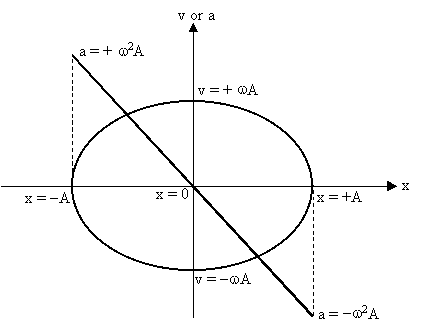### Energy

• In an ideal system with no drag forces, energy is conserved:
$E_K + E_P = E_{total}\text{ remains constant.}$
• The total energy of a system can be expressed in terms of the maximum kinetic energy of the system:
$E_{total} = \frac{1}{2} mv_{max}^2 = \frac{1}{2}mA^2\omega^2$
• So kinetic and potential energy are:
$E_{K} = \frac{1}{2} mv^2 = \frac{1}{2}m\omega^2( A^2 \cos^2(\omega t + \phi))\\[1em] = \frac{1}{2}m\omega^2( A^2 (1 - \sin^2(\omega t + \phi)))\\[1em] = \frac{1}{2}m\omega^2 (A^2 - x^2)\\[2em] E_P = E_{total} - E_K= \frac{1}{2}mA^2\omega^2 -\frac{1}{2}m\omega^2 (A^2 - x^2)\\[1em] = \frac{1}{2}m\omega^2x^2$
• note that $v^2 = A^2 \cos^2(\omega t + \phi)$ and $x ^2 = A^2\sin^2(\omega t + \phi)$.## Single slit diffraction

• For destructive interference, the path difference must be $(n + \frac{1}{2})\lambda$, and the first minimum is obtained where path difference = $\frac{1}{2}\lambda$.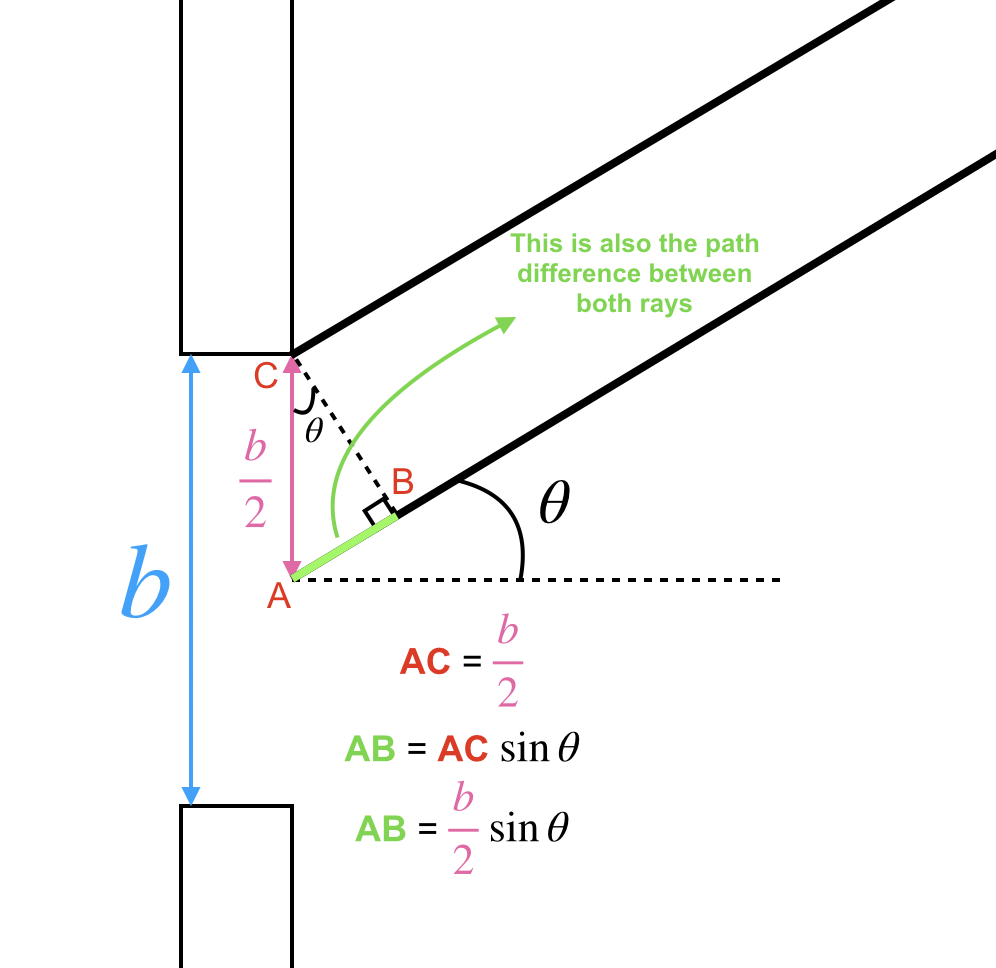• With the aid of the diagram, we know that AB is the path difference between the two waves, the slit width is $b$, and $\lambda$ is the wavelength:
\begin{aligned} \text{AB} &= \text{path difference} \\ \text{path difference} &= \frac{1}{2}\lambda \text{ (for the first minimum)} \\ \text{AB} &= \frac{1}{2}\lambda \\ \text{AB} &= \frac{b}{2}\sin\theta \text{ (using trignometry)} \\ \frac{1}{2}\lambda &= \frac{b}{2}\sin\theta \\ b\sin\theta &= \lambda \\ \sin\theta &= \frac{\lambda}{b} \\ \text{Since } \sin\theta &\approx \theta \text{ for very small angles:} \\ \theta &= \frac{\lambda}{b} \end{aligned}
• So, we can say that the first minimum for a single slit diffraction is observed at an angle $\theta$ where $\theta = \frac{\lambda}{b}$.
• The formula to find the angle at which additional minima form becomes:
$b\sin\theta = n\lambda$
• Where:
• $b$ : slit width.
• $n$: the nth minima.
• $\lambda$: wavelength.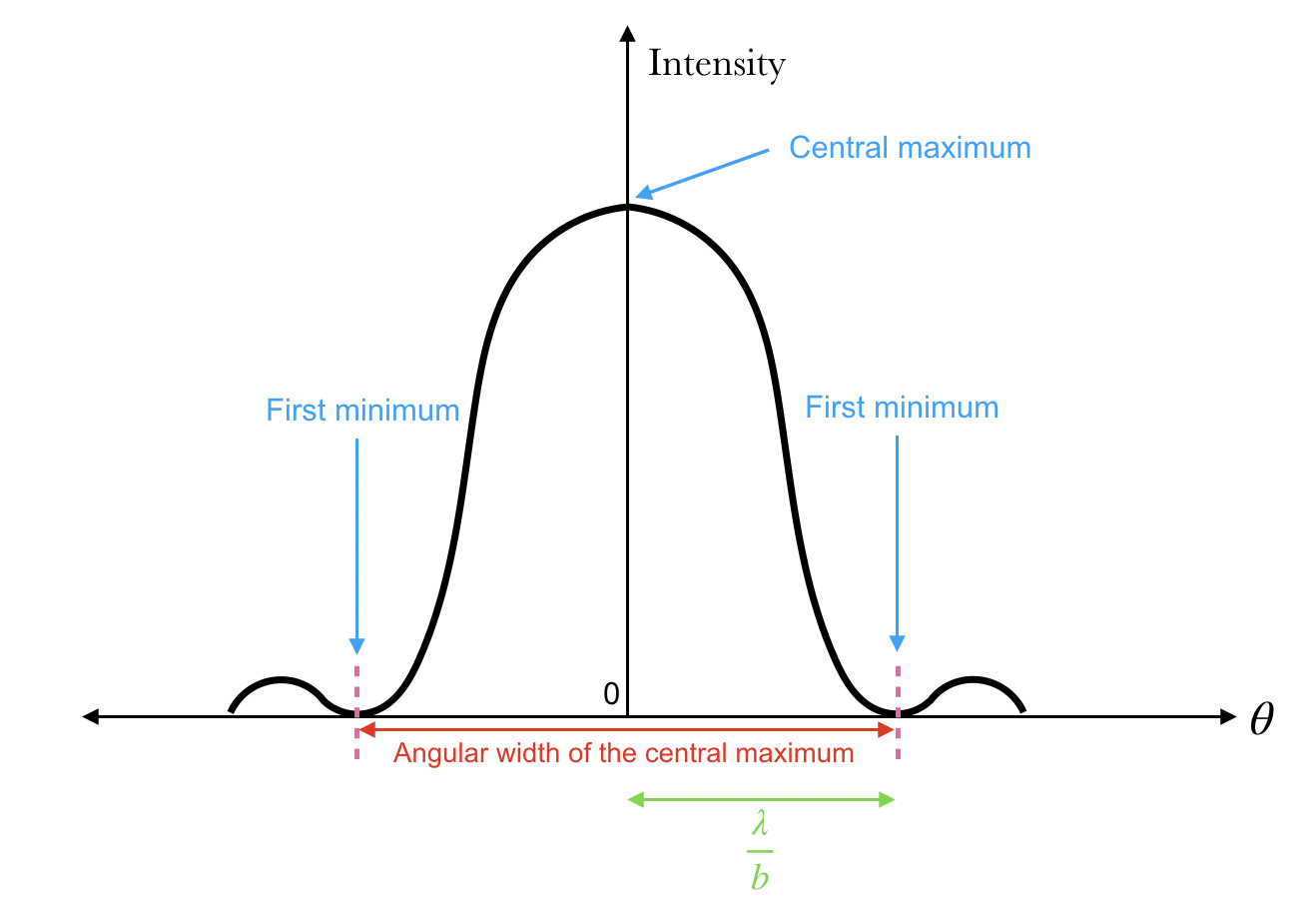• We can also conclude that:
$\theta \propto \lambda$
• As wavelength $\uparrow$, $\theta \uparrow$, and the angular width of the central maxima $\uparrow$.
$\theta \propto \frac{1}{b}$
• As $b \uparrow$, $\theta \downarrow$, and so the angular width of the central maxima $\downarrow$.

## Young’s Double Slit Experiment

• When light from two slits intefere in the following setup, a set of fringes are formed:
•• The following conditions are required:
• The light from both slits must be coherent, ie, constant or zero phase difference.
• The distance of between the slits and the slit width must be negligible compared to the distance between the screen and the slits.
• The slit width should be comparable to the wavelength of the light for circular diffraction.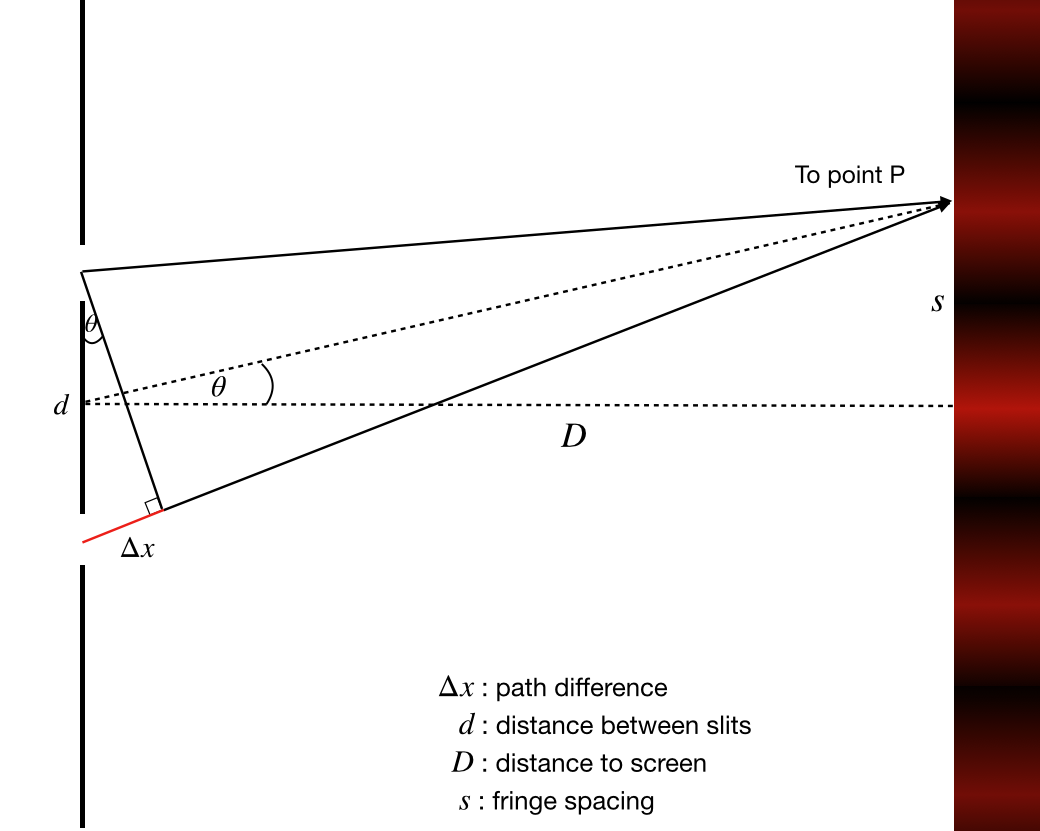• Since $D >> d$, it can be assumed that the two rays are parallel.
• For constructive interference at point $P$, $\Delta x = n\lambda$, where $n = 0, 1, 2, 3, 4, \dots$.
• In this case, $n = 1$.
• $\Delta x = \lambda = d\sin\theta$
• $\tan\theta = \frac{s}{D}$
• We can assume $\tan\theta = \sin\theta$ because $\theta$ is extremely small.
• $\lambda = d (\tan \theta ) = \frac{ds}{D}$
• Finally, we can conclude that:
• $s = \frac{\lambda D}{d}$

The graph of light intensity vs angle from center for both single and double slit diffraction is as follows:• The intensity of the double slit pattern is modulated by the one-slit pattern.

### Multi-slit diffraction

• If the number of slits are increased (with the same length between each slit), the fringes are more distinctly pronounced:• For $N$ slits, there are $N - 2$ secondary maxima between two primary maxima.
• With an increase in the number of slits to $N$:
• the primary maxima will become thinner and sharper
• The $N - 2$ secondary maxima will become unimporant
• The intensity of the central maximum is proportional to $N^2$.

## Diffraction grating

• used in spectroscopy to measure the wavelength of light.
• Has rulings, which are slits, which help determine the slit seperation.
• $x$ lines/rulings per millimetre corresponds to a $\frac{1}{x}$ slit seperation.
• $d = \frac{1}{x}$
• Since we know that the condition for constructive interference is $d\sin\theta = n\lambda$, we can use this to calculate the wavelength of light.

## Thin-film interference

• Upon reflection on a surface with a refractive index greater than the medium a ray is already in, the ray undergoes a phase change of $\pi$.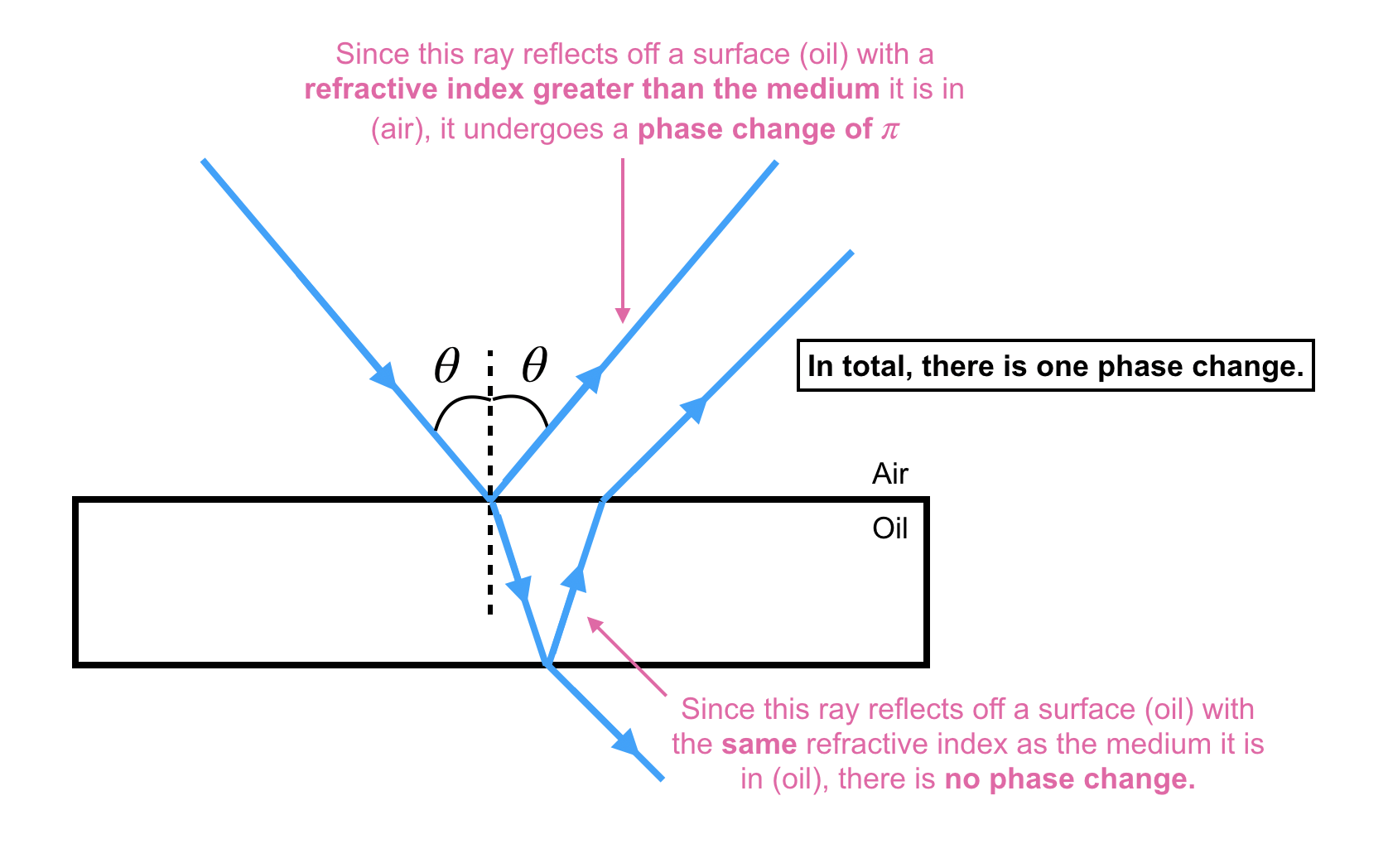• If $d$ is the thickness of the film, the condition for constructive interference, when only one phase change occurs (light is reflected off a medium with a greater refractive index only once):
$2dn = (m + \frac{1}{2})\lambda$
• Where:
• $d$ : thickness of the film.
• $n$ : refractive index.
• $m$ : an integer.

Note: It is similar to the condition for destructive interference ($n+\frac{1}{2}\lambda$), but it is the condition for constructive interference in this case because of the phase shift of $\pi$, resulting in crests becoming troughs and troughs becoming crests.

• The condition for destructive interference, when only one phase change occurs(light is reflected off a medium with a greater refractive index only once):
$2dn = m\lambda$
• The condition for constructive interference when there is no or two phase changes (light is reflected off a medium with a greater refractive index twice):
$2dn = m\lambda$
• The condition for destructive interference when there is no or two phase changes (light is reflected off a medium with a greater refractive index twice):
$2dn = (m + \frac{1}{2})\lambda$

## Resolution

• The angular separation of two objects is given by $\theta_A=\frac{s}{D}$ where $s$ is the distance between the objects, and $D$ is the distance between the observer and the objects.
• According to the Rayleigh criterion, resolution is possible when the angular separation is greater than the angle fo the first diffraction minimum: $\theta_A \geq \frac{\lambda}{b}$
• For a circular slit, the following criteria is used: $\theta_A \geq 1.22\frac{\lambda}b$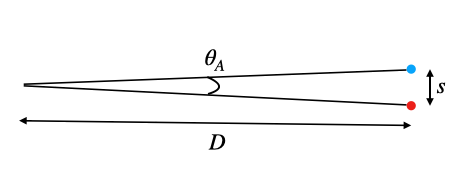### In a diffraction grating

$R = \frac{\lambda_{avg}}{\Delta \lambda} = m N$
where:

• $R$: resolving power of the grating
• $\lambda_{avg}$: The average of the two wavelengths to be resolved
• $\Delta \lambda$: difference in the wavelengths that are to be resolved
• $m$:

## Doppler Effect

• The doppler effect is the change in the observed frequency of a wave which happens whenever there is relative motion between the source and the observer.
• If the wavelength of the light decreases, the source is moving towards the observer, which is called a blueshift.
• If the wavelength of the light increases, the source is moving away from the observer, which is called a redshift.
• If a source is moving towards an observer with velocity $u_s$:
$\lambda' = \lambda(1- \frac{u_s}{v})\\[1.5em] f' = f(\frac{v}{v - u_s})$
• If an observer is moving towards a source with velocity $u_o$:
$f' = f(\frac{v+u_o}{v})$

### For light

• If the speed of the observer is small compared to the speed of light, then: $\frac{\Delta f}{f} \approx \frac{v}{c}\\[1.5em]\frac{\Delta \lambda}{\lambda} \approx \frac{v}{c}$
• K. A. Tsokos - Physics for the IB Diploma, Sixth Edition
• Class Notes